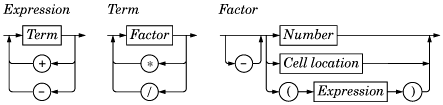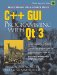# Subclassing QTableItem

The Cell class inherits from QTableItem. The class is designed to work well with Spreadsheet, but it has no specific dependencies on that class and could in theory be used in any QTable.

```#ifndef CELL_H
#define CELL_H

#include
#include

class Cell : public QTableItem
{
public:
Cell(QTable *table, const QString &formula);

void setFormula(const QString &formula);
QString formula() const;
void setDirty();
QString text() const;
int alignment() const;

private:
QVariant value() const;
QVariant evalExpression(const QString &str, int &pos) const;
QVariant evalTerm(const QString &str, int &pos) const;
QVariant evalFactor(const QString &str, int &pos) const;

QString formulaStr;
mutable QVariant cachedValue;
mutable bool cacheIsDirty;
};

#endif
```

The Cell class extends QTableItem by adding three private variables:

• formulaStr stores the cell's formula as a QString.
• cachedValue caches the cell's value as a QVariant.
• cacheIsDirty is true if the cached value isn't up to date.

The QVariant type can hold values of many C++ and Qt types. We use it because some cells have a double value, while others have a QString value.

The cachedValue and cacheIsDirty variables are declared with the C++ mutable keyword. This allows us to modify these variables in const functions. Alternatively, we could recalculate the value each time text() is called, but that would be needlessly inefficient.

Notice that there is no Q_OBJECT macro in the class definition. Cell is a plain C++ class, with no signals or slots. In fact, because QTableItem doesn't inherit from QObject, we cannot have signals and slots in Cell as it stands. Qt's item classes don't inherit from QObject to keep their overhead to the barest minimum. If signals and slots are needed, they can be implemented in the widget that contains the items or, exceptionally, using multiple inheritance with QObject.

Here's the start of cell.cpp:

```#include
#include

#include "cell.h"

Cell::Cell(QTable *table, const QString &formula)
: QTableItem(table, OnTyping)

{
setFormula(formula);
}
```

The constructor accepts a pointer to a QTable and a formula. The pointer is passed on to the QTableItem constructor and is accessible afterward as QTableItem::table(). The second argument to the base class constructor, OnTyping, means that an editor pops up when the user starts typing in the current cell.

```void Cell::setFormula(const QString &formula)
{
formulaStr = formula;
cacheIsDirty = true;
}
```

The setFormula() function sets the cell's formula. It also sets the cacheIsDirty flag to true, meaning that cachedValue must be recalculated before a valid value can be returned. It is called from the Cell constructor and from Spreadsheet::setFormula().

``` QString Cell::formula() const
{
return formulaStr;
}
```

The formula() function is called from Spreadsheet::formula().

```void Cell::setDirty()
{
cacheIsDirty = true;
}
```

The setDirty() function is called to force a recalculation of the cell's value. It simply sets cacheIsDirty to true. The recalculation isn't performed until it is really necessary.

```QString Cell::text() const
{
if (value().isValid())
return value().toString();
else
return "####";
}
```

The text() function is reimplemented from QTableItem. It returns the text that should be shown in the spreadsheet. It relies on value() to compute the cell's value. If the value is invalid (presumably because the formula is wrong), we return "####".

The value() function used by text() returns a QVariant. A QVariant can store values of different types, such as double and QString, and provides functions to convert the variant to other types. For example, calling toString() on a variant that holds a double value produces a string representation of the double. A QVariant constructed using the default constructor is an "invalid" variant.

```int Cell::alignment() const
{
if (value().type() == QVariant::String)
return AlignLeft | AlignVCenter;
else
return AlignRight | AlignVCenter;
}
```

The alignment() function is reimplemented from QTableItem. It returns the alignment for the cell's text. We have chosen to left-align string values and to right-align numeric values. We vertically center all values.

```const QVariant Invalid;
QVariant Cell::value() const
{
if (cacheIsDirty) {
cacheIsDirty = false;

if (formulaStr.startsWith("'")) {
cachedValue = formulaStr.mid(1);
} else if (formulaStr.startsWith("=")) {
cachedValue = Invalid;
QString expr = formulaStr.mid(1);
expr.replace(" ", "");
int pos = 0;
cachedValue = evalExpression(expr, pos);
if (pos < (int) expr.length())
cachedValue = Invalid;
} else {
bool ok;
double d = formulaStr.toDouble(&ok);
if (ok)
cachedValue = d;
else
cachedValue = formulaStr;
}
}
return cachedValue;
}
```

The value() private function returns the cell's value. If cacheIsDirty is true, we need to recalculate the value.

If the formula starts with a single quote (for example, "'12345"), the value is the string from position 1 to the end. (The single quote occupies position 0.)

If the formula starts with '=', we take the string from position 1 and delete any spaces it may contain. Then we call evalExpression() to compute the value of the expression. The pos argument is passed by reference; it indicates the position of the character where parsing should begin. After the call to evalExpression(), pos is equal to the length of the expression that was successfully parsed. If the parse failed before the end, we set cachedValue to be Invalid.

If the formula doesn't begin with a single quote or an equals sign ('='), we attempt to convert it to a floating point value using toDouble(). If the conversion works, we set cachedValue to be the resulting number; otherwise, we set cachedValue to be the formula string. For example, a formula of "1.50" causes toDouble() to set ok to true and return 1.5, while a formula of "World Population" causes toDouble() to set ok to false and return 0.0.

The value() function is a const function. We had to declare cachedValue and cacheIsValid as mutable variables so that the compiler will allow us to modify them in const functions. It might be tempting to make value() non-const and remove the mutable keywords, but that would not compile because we call value() from text(), a const function. In C++, caching and mutable usually go hand in hand.

We have now completed the Spreadsheet application, apart from parsing formulas. The rest of this section covers evalExpression() and the two helper functions evalTerm() and evalFactor(). The code is a bit complicated, but it is included here to make the application complete. Since the code is not related to GUI programming, you can safely skip it and continue reading from Chapter 5.

The evalExpression() function returns the value of a spreadsheet expression. An expression is defined as one or more terms separated by '+' or '-' operators; for example, "2*C5+D6" is an expression with "2*C5" as its first term and "D6" as its second term. The terms themselves are defined as one or more factors separated by '*' or '/' operators; for example, "2*C5" is a term with "2" as its first factor and "C5" as its second factor. Finally, a factor can be a number ("2"), a cell location ("C5"), or an expression in parentheses, optionally preceded by a unary minus. By breaking down expressions into terms and terms into factors, we ensure that the operators are applied with the correct precedence.

The syntax of spreadsheet expressions is defined in Figure 4.12. For each symbol in the grammar (Expression, Term, and Factor), there is a corresponding Cell member function that parses it and whose structure closely follows the grammar. Parsers written this way are called recursive-descent parsers.

Figure 4.12. Syntax diagram for spreadsheet expressions```QVariant Cell::evalExpression(const QString &str, int &pos) const
{
QVariant result = evalTerm(str, pos);
while (pos < (int)str.length()) {
QChar op = str[pos];
if (op != '+' && op != '')
return result;
++pos;

QVariant term = evalTerm(str, pos);
if (result.type() == QVariant::Double
&& term.type() == QVariant::Double) {
if (op == '+')
result = result.toDouble() + term.toDouble();
else
result = result.toDouble() - term.toDouble();
} else {
result = Invalid;
}
}
return result;
}
```

First, we call evalTerm() to get the value of the first term. If the following character is '+' or '-', we continue by calling evalTerm() a second time; otherwise, the expression consists of a single term, and we return its value as the value of the whole expression. After we have the value of the first two terms, we compute the result of the operation, depending on the operator. If both terms evaluated to a double, we compute the result as a double; otherwise, we set the result to be Invalid.

We continue like this until there are no more terms. This works correctly because addition and subtraction are left-associative; that is, "123" means "(12)3", not "1(23)".

```QVariant Cell::evalTerm(const QString &str, int &pos) const
{
QVariant result = evalFactor(str, pos);
while (pos < (int)str.length()) {
QChar op = str[pos];
if (op != '*' && op != '/')
return result;
++pos;

QVariant factor = evalFactor(str, pos);
if (result.type() == QVariant::Double
&& factor.type() == QVariant::Double) {
if (op == '*') {
result = result.toDouble() * factor.toDouble();
} else {
if (factor.toDouble() == 0.0)
result = Invalid;
else
result = result.toDouble() / factor.toDouble();
}
} else {
result = Invalid;
}
}
return result;
}
```

The evalTerm() function is very similar to evalExpression(), except that it deals with multiplication and division. The only subtlety in evalTerm() is that we must avoid division by zero. While it is generally inadvisable to test floating point values for equality because of rounding errors, it is safe to do so to prevent division by zero.

```QVariant Cell::evalFactor(const QString &str, int &pos) const
{
QVariant result;
bool negative = false;
if (str[pos] == '') {
negative = true;
++pos;
}

if (str[pos] == '(') {
++pos;
result = evalExpression(str, pos);
if (str[pos] != ')')
result = Invalid;
++pos;
} else {
QRegExp regExp("[AZaz]   {0,2}");
QString token;

while (str[pos].isLetterOrNumber() || str[pos] == '.') {
token += str[pos];
++pos;
}

if (regExp.exactMatch(token)) {
int col = token.upper().unicode()  'A';
int row = token.mid(1).toInt()  1;

Cell *c = (Cell *)table()->item(row, col);
if (c)
result = c->value();
else
result = 0.0;
} else {
bool ok;
result = token.toDouble(&ok);
if (!ok)
result = Invalid;
}
}

if (negative) {
if (result.type() == QVariant::Double)
result = -result.toDouble();
else
result = Invalid;
}
return result;
}
```

The evalFactor() function is a bit more complicated than evalExpression() and evalTerm(). We start by noting whether the factor is negated. We then see if it begins with an open parenthesis. If it does, we evaluate the contents of the parentheses as an expression by calling evalExpression(). This is where recursion occurs in the parser; evalExpression() calls evalTerm(), which calls evalFactor(), which calls evalExpression() again.

If the factor isn't a nested expression, we extract the next token, which may be a cell location or a number. If the token matches the QRegExp, we take it to be a cell reference and we call value() on the cell at the given location. The cell could be anywhere in the spreadsheet, and it could have dependencies on other cells. The dependencies are not a problem; they will simply trigger more value() calls and (for "dirty" cells) more parsing until all the dependent cell values are calculated. If the token isn't a cell location, we take it to be a number.

What happens if cell A1 contains the formula "=A1"? Or if cell A1 contains "=A2" and cell A2 contains "=A1"? Although we have not written any special code to detect circular dependencies, the parser handles these cases gracefully by returning an invalid QVariant. This works because we set cacheIsDirty to false and cachedValue to Invalid in value() before we call evalExpression(). If evalExpression() recursively calls value() on the same cell, it returns Invalid immediately, and the whole expression then evaluates to Invalid.

We have now completed the formula parser. It would be straightforward to extend it to handle predefined spreadsheet functions, like "sum()" and "avg()", by extending the grammatical definition of Factor. Another easy extension is to implement the '+' operator with string operands (as concatenation); this requires no changes to the grammar.C++ GUI Programming with Qt 3
ISBN: 0131240722
EAN: 2147483647
Year: 2006
Pages: 140